# Spin-Spin Coupling

| Home | | Organic Chemistry |

## Chapter: Organic Chemistry : Structure Determination of Organic Compounds

The determination of the numbers of different kinds protons in a molecule is a very important use of NMR spectroscopy, but it does not establish the connectivity of the carbons bearing those protons and the connectivity is crucial in correct structure determination.

SPIN-SPIN COUPLING

The determination of the numbers of different kinds protons in a molecule is a very important use of NMR spectroscopy, but it does not establish the connectivity of the carbons bearing those protons and the connectivity is crucial in correct structure determination. However, NMR spectroscopy can also give insight into the connections between functional groups by the presence of spin – spin coupling in the NMR spectrum.

Consider the NMR spectrum of 1,1-dichloro-2,2-dibromoethane (Figure 11.11). Based on the different electronegativities of chlorine and bromine, the two protons in the molecule are nonequivalent and should thus give signals at different chemical shifts with the same integrated areas. The dichloromethyl proton should appear downfield relative to the dibromomethyl proton. The actual NMR spectrum indeed shows two different signals, one for Ha and one for Hb , but each absorption consists of two lines and is termed a doublet. The signal for each proton is thus “split” into two resonances. This splitting is due to the fact that each proton can sense the spin state of the neighboring proton and is called spin – spin splitting.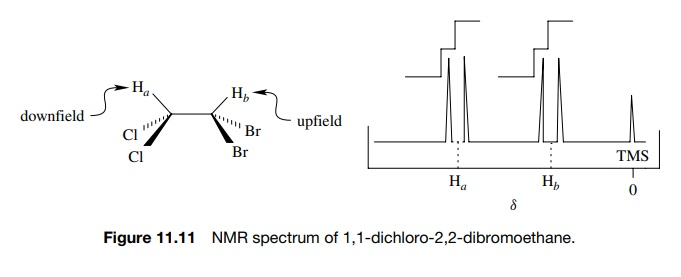In the magnetic field of the NMR spectrometer, both Ha and Hb have distinct absorption frequencies based on the Heff each proton experiences. This gives rise to individual signals for Ha and Hb . Focus now on one hydrogen, Ha . The hydrogen which is next to Ha (namely Hb ) has two spin states (aligned or opposed to Ho) that are nearly equally populated (actually there are slightly more in the lower energy spin state than the upper, but the difference is very small). Thus the magnetic moment of the neighboring proton Hb (µHb ) either adds or subtracts an incremental amount (µHb ) to Heff — the field experienced by Ha . As a consequence Ha will experience two distinct magnetic fields Heff µHb and Heff + µHb . Consequently Ha will absorb energy at two distinct frequencies, and the signal for Ha will be split into two lines— a doublet— due to the presence of the adjacent proton Hb .Focusing now on Hb , the same analysis leads to the prediction that Hb will also experience two distinct magnetic fields, Heff µHa and Heff + µHa ; absorb energy at two different frequencies; and thus will be split into a doublet by the presence of Ha (Figure 11.12).

The middle of the doublet corresponds to the actual chemical shift of the proton due to Heff, the total integrated area under both lines of the doublet corresponds to the signal intensity of one proton, and the width between the two lines in hertz (cps) is called J , the coupling constant. The coupling constant is a measure of the strength of the interaction between the coupled nuclei that leads to spin – spin splitting. The J values for proton – proton coupling can range from 0 to 20 Hz, but most commonly coupling constants fall in the range of 0 – 10 Hz.

A value of J = 0 means that there is no significant interaction with neigh-boring protons, and thus the absorption is not affected by the spin states of neighboring protons. This normally occurs when there are more than three bonds separating different types of protons.Geminal coupling (two bonds, Jgem) and vicinal coupling (three bonds, Jvic) are the types of spin – spin splitting normally encountered. In addition, the interaction between protons is reciprocal— if two protons are coupled, they are coupled equally and J1,2 = J2,1. That is, if Ha is split by Hb by some amount, say J = 6 Hz, then Hb must be split by Ha by J = 6 Hz. Finally equivalent protons do not split each other; thus the t -butyl hydrogens of t -butanol are a singlet (Figure 11.13).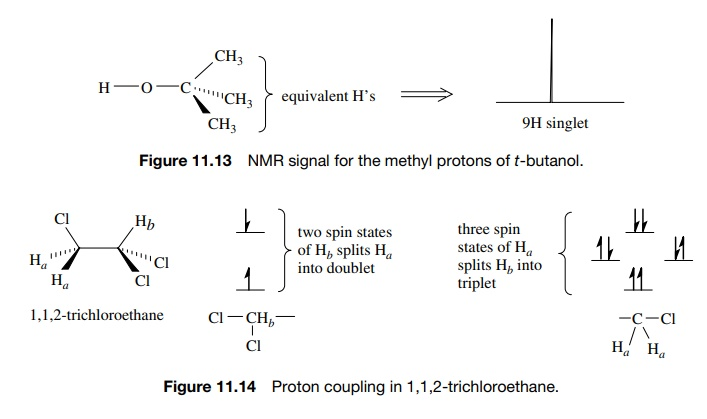If there are more than one neighboring hydrogen atom, then different split-ting patterns are observed. For example, 1,1,2-trichloroethane has two signals, a two-proton doublet (J = 6 Hz) upfield (Ha ) and a one-proton triplet (J = 6 Hz) downfield (Hb ) (Figure 11.14). The equivalent protons Ha of the CH2–Cl group give the upfield absorption, which is split into a doublet by the single vicinal proton Hb . The single methine proton Hb gives the downfield absorption, which is split into a triplet by the two adjacent, equivalent methylene protons Ha . The triplet splitting is due to the three spin distributions possible for the two equiv-alent CH2 protons, both aligned, one aligned (two possibilities), both opposed. The Hb triplet has three lines in a 1 : 2 : 1 ratio, which reflects the numbers of spin states of the neighboring CH2 group. Because the two sets of protons are coupled, the spacing between each line of the triplet (J = 6 Hz) must be the same as the doublet splitting (J = 6 Hz). The middle line of the triplet corresponds to the chemical shift of the CH2 group, whereas the middle of the doublet corresponds to the chemical shift of the methine proton Hb (Figure 11.15).Diethyl ether (Figure 11.16) has a three-proton triplet at 1.2δ (J = 7 Hz) for the methyl protons, which are split by the two protons of the CH2 group. The methylene protons absorb at 3.3δ and are split into four lines (quartet) in a 1 : 3 : 3 : 1 ratio (Figure 11.17). This splitting occurs because the three equiv-alent protons of the methyl group can have four possible spin distributions which are nearly equally populated. They are three aligned; two aligned, one opposed (three possibilities); one aligned, two opposed (three possibilities); and all opposed. The center of the quartet is the chemical shift of the CH2 group and the coupling constant of the quartet (J = 7 Hz) must be the same as the coupling constant of the methyl triplet (J = 7 Hz) since the two sets of protons are coupled. (When protons are coupled, the signal for each set is split by the same coupling constant.)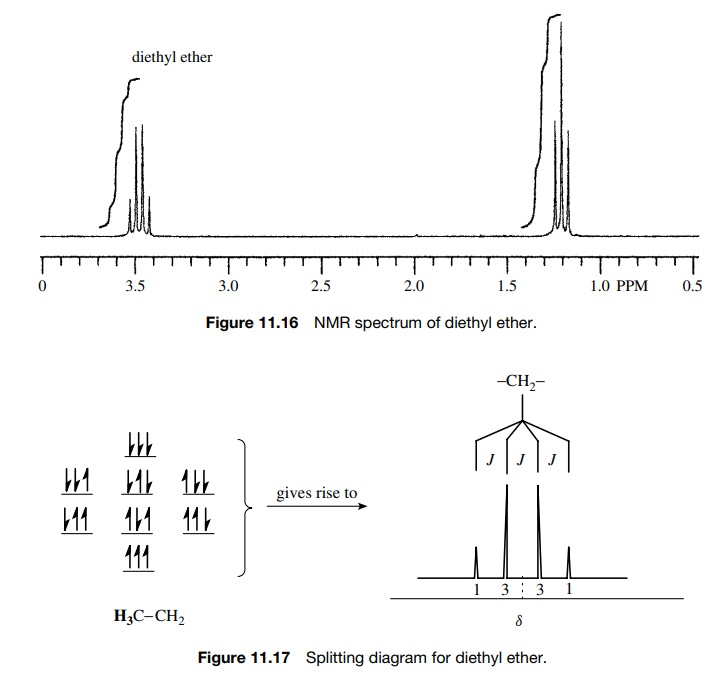If these considerations are generalized, it is seen that the signals for protons coupled equally to n equivalent vicinal protons will be split in to multiplets having n + 1 lines. The intensities of the individual lines in the multiplets follow

Pascal’s triangle:The middle of the multiplet is the chemical shift of the protons responsible for that absorption, and the total integrated area under the multiplet corresponds to the total number of protons of the signal; however, the integrated area of the individual lines of the multiplet are in the ratio of Pascal’s triangle. Several examples of simple splitting patterns are shown: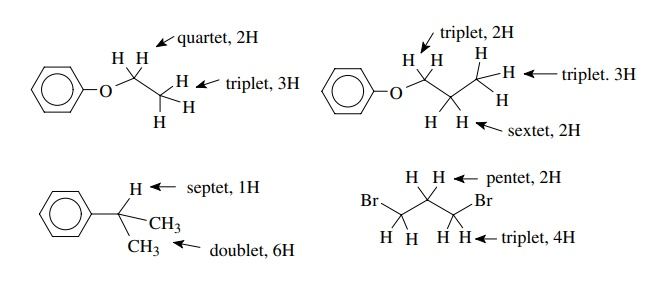The n + 1 rule for predicting the multiplicity of a given proton signal holds when the coupling constants with all of the nearest neighbors are the same. For example, the multiplicity of the central methylene group of 1-bromo-3-chloropropane (Figure 11.18) is a pentet which requires that J12 = J23. That is, the central methylene group has the same coupling constant to the protons of the bromomethyl group (J12) as to the protons of the chloromethyl group (J23). Those groups are not equivalent and have different chemical shifts, but each signal is split into a triplet by the C-2 methylene group by the same J value.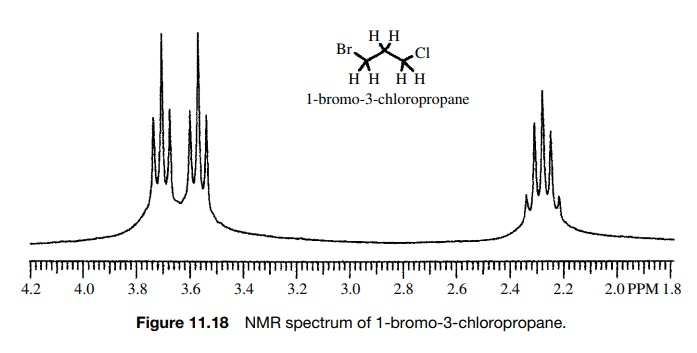If a proton or set of protons is not coupled equally to neighboring protons, then the n + 1 rule is not adequate to describe the multiplicity of the absorption. Instead one observes multiplets of multiplets as the splitting pattern (e.g., doublet of doublets or triplet of doublets). The multiplicity can be understood by carrying out sequential splitting diagrams. For example, consider a proton Hb split by two neighboring vicinal protons Ha and Hc by Jab = 2 Hz and Jbc = 7 Hz. This is shown schematically in Figure 11.19 where the Hb signal is split into a doublet by Hc (Jbc = 7 Hz) and each line of that doublet is split into a doublet by Ha (Jab = 2 Hz).The result is a doublet of doublets. The spacing between the small doublet splitting is J = 2 Hz and the splitting between the centers of the two doublets is J = 7 Hz. The same diagram is produced by first splitting the Hb signal by Jab = 2 Hz and then splitting each line into a doublet by Jbc = 7 Hz. Because of the requirement that Jab = Jba , Ha will be split into a doublet (J =  2 Hz) by Hb and Hc will also be split into a doublet (J = 7 Hz) by Hb . Taking into account these different splitting patterns, the connectivity relationships between Ha , Hb , and Hc are clear. Because Ha and Hc are both doublets but they are split by different coupling constants, they cannot be coupled to each other. The signal for Hb , however, is seen to be a doublet of doublets with J = 2 Hz and J = 7 Hz. Since these values are the same as the couplings of Ha and Hc , Hb is coupled to both Ha and Hc and the connectivity is thus between Ha , Hb , and Hc . Splitting patterns are thus powerful ways to establish connectivity in molecules. The patterns seen in Figures 11.20 and 11.21 are typical of the types of connections encountered in various organic compounds.